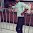# In load flow analysis, the load connected at a bus is represented as

In load flow analysis, the load connected at a bus is represented as
1. the constant current drawn from the bus
2. constant impedance connected at the bus
3. voltage and frequency dependent source at the bus
4. constant real and reactive drawn from the bus
Answer: Constant real and reactive drawn from the bus

Load flow studies determine if system voltages remain within specified limits under normal or emergency operating conditions and whether equipment such as transformers and conductors are overloaded. Load flow studies are commonly used to Optimise component or circuit loading. Develop practical bus voltage profiles.
• Load flow study is the steady state analysis of power system network.
• Load flow solves a set of simultaneous non-linear algebraic power equations for the two unknown variables (|V| and ∠δ ) at each node in a system.
• To solve non-linear algebraic equations it is important to have fast, efficient and accurate numerical algorithms.
• The output of the load flow analysis is the voltage and phase angle, real and reactive power (both sides in each line), line losses and slack bus power.←Previous
« Prev Post
Next→
Next Post »

#### 1 comment:

1.Thanks for helping me.